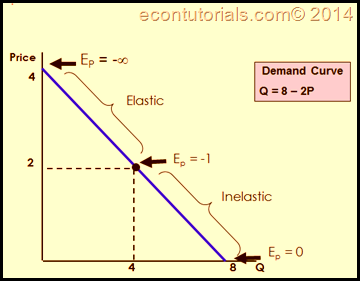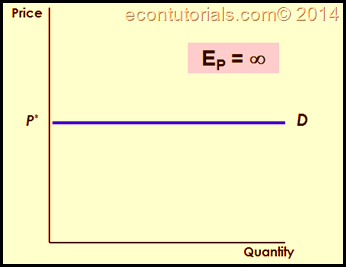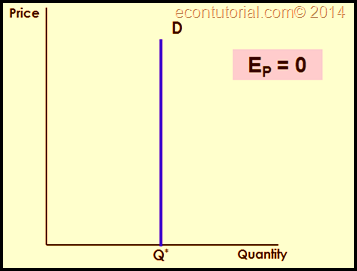Select Page

We described one of the most important concerning topics of Principles of Microeconomics that is ELASTICITY . But what happened as we move along a demand curve ? Since its downward Sloping, what consequences does it has for the elasticity that we just studied?  Here comes comes the part where we need to know that Elasticity is so very much affected by the Slope as well as the Price and Quantity . And it changes along the Demand Curve.  Let me show that Graphically first.## Interpretation

• The basic Reason for Using a linear Demand Curve is because it can clearly show the Effect over Elasticity. Also because the slope is constant for a Linear Demand Curve.
• With this graph we can analyze t hat Elasticity does depends up the Slope as well as on the Values of Q and P.
• Now the Graph shows that the upper portion is Elastic which means that a Slight change in Price will change the Quantity rapidly or Aggressively you can say
• The lower portion shows a constant or Equal Change which interprets that the percentage change in price either higher or lower will exactly bring the same percentage change in Quantity.
• The lower portion shows Inelasticity. In this Scenario a change in Price of a product would not effect the demand for that Good. The values in this portion will be <1.
• We can conclude that the elasticity becomes smaller or less as we move down along the curve.

We can also interpret the curve in the following way :

• If the Curve is going steep or steeper, the demand for the good will be inelastic.
• If the Curve is going Flat or Flatter, the demand for the good will be Elastic.

## Two Extreme Cases

We arrive here at a question:

• What if the Demand curve is Completely Steeper or Flatter ? How will this effect the Elasticity ?
1. Infinitely Elastic Demand—>Horizontal
2. Completely Inelastic Demand—>Vertical

Graphically:The First graph on the Left shows Infinitely Elastic Demand. Which means that a Slight change in Price would Great or you can say highly effect the Demand for that Product. You will find such cases in Perfect Competition which we will be discussing in our later topics.

The other Graph is Completely Inelastic Demand which shows that No matter what the price be, either very high or low the consumers will demand for the product. Although Oil and Wheat are such resources whose example we can take but of course its no Completely Inelastic. Somehow in the long run we do find its substitutes (Gas, etc). (Long Run ? More on that Later).

Next is Other Demand Elasticities and Short-run versus Long-run…

Shares